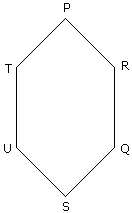# Verbal Reasoning - Seating Arrangement

Six friends P, Q, R, S, T and U are sitting around the hexagonal table each at one corner and are facing the centre of the hexagonal. P is second to the left of U. Q is neighbour of R and S. T is second to the left of S.
1.

Which one is sitting opposite to P ?

 A. R B. Q C. T D. S

Explanation:S is sitting opposite to P.

2.

Who is the fourth person to the left of Q ?

 A. P B. U C. R D. Data inadequate

Explanation:P is the fourth person to the left of Q.

3.

Which of the following are the neighbours of P ?

 A. U and P B. T and R C. U and R D. Data inadequate

Explanation:T and R are the neighbours of P.

4.

Which one is sitting opposite to T ?

 A. R B. Q C. Cannot be determined D. S